#2019 iT 邦幫忙鐵人賽DAY 4
4
AI & Data

## Day04 幾何資料基本運算

GeoDataFrame使操作GIS資料分析時更有彈性

• 坐標轉換
• 幾何操作
• 空間運算子

## 坐標轉換

Geopandas依賴pyrpoj，proj是坐標轉換非常方便的工具，

``````import geopandas as gpd
gdf_Lib.crs = {'init' :'epsg:4326'} # 避免資料沒設，這邊再重新給一次
gdf_Lib=gdf_Lib.to_crs(epsg=3826)
gdf_Lib
``````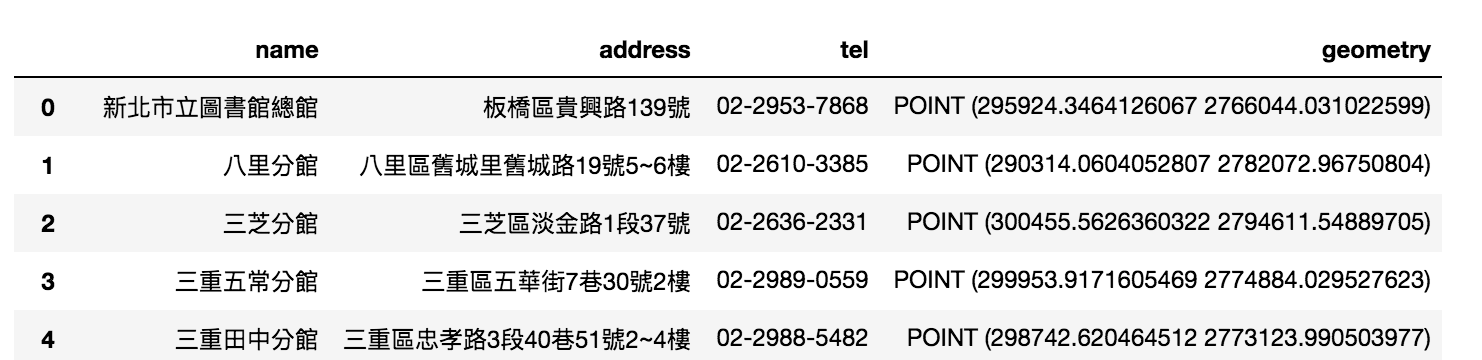## 幾何操作

Geopandas的幾何操作主要是來自shaply套件，以下來試看看在GIS軟體常用的功能

### buffer

buffer在GIS中常用來分析點線面的影響範圍，

``````base=gdf_Lib.head(1).buffer(100).plot()

``````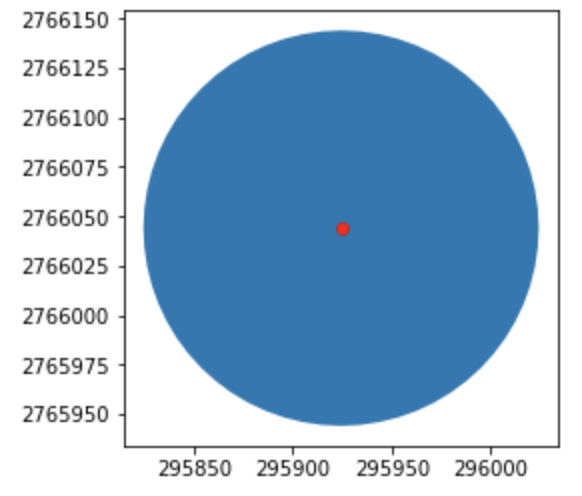### area

area是GeoDataFrame中，提供每一筆幾何資料的面積

``````buffer=gdf_Lib.head(1).buffer(100)
area=buffer.area
print(area)

### # 0 31365.4849055
``````

### envelope

envelope是整個GeoDataFrame中，每一筆資料包覆的`長方形`範圍，它是一個四角坐標

``````envelope=buffer.envelope
print(envelope)

# 0    POLYGON ((295824.3464126067 2765944.031022599,...

``````

### convex_hull

convex hull與envelope類似但不一樣，它是包住每一個資料的凸殼多邊形

``````convexhull=buffer.convex_hull
print(convexhull)

### 0    POLYGON ((295924.3464126067 2765944.031022599,...
``````

``````base=envelope.plot()
convexhull.plot(ax=base,color='brown')

``````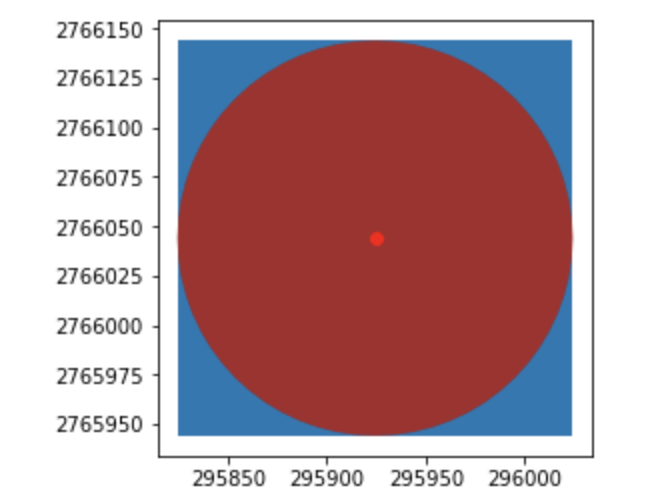### 幾何轉換

Geodataframe可以進行幾何的投影轉換，

``````base=buffer.scale(10,5).plot(color='yellow')
buffer.plot(ax=base,color='brown')
``````

The Shapely User Manual — Shapely 1.2 and 1.3 documentation

## 空間運算子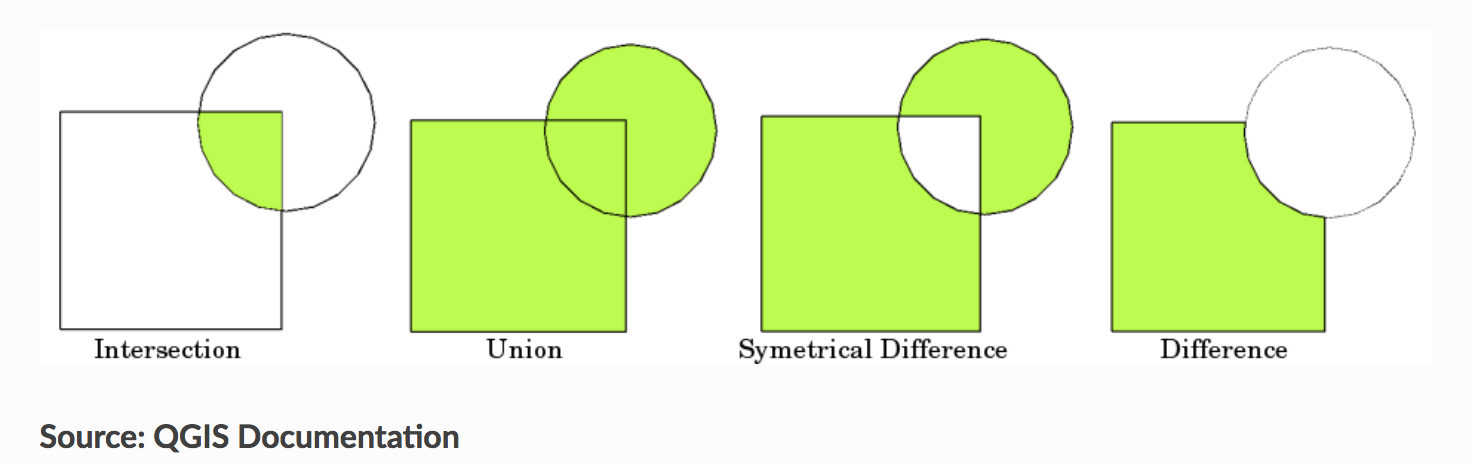[取自Geopandas/QGIS]

``````p1=gpd.read_file('output/Library.shp',encoding='utf-8').head(1)
p1.crs = {'init' :'epsg:4326'} # 避免資料沒設，這邊再重新給一次
p1=p1.to_crs(epsg=3826)
p1['geometry']=p1.buffer(30).translate(xoff=20.0, yoff=0.0)

p2.crs = {'init' :'epsg:4326'} # 避免資料沒設，這邊再重新給一次
p2=p2.to_crs(epsg=3826)
p2['geometry']=p2.buffer(30)
``````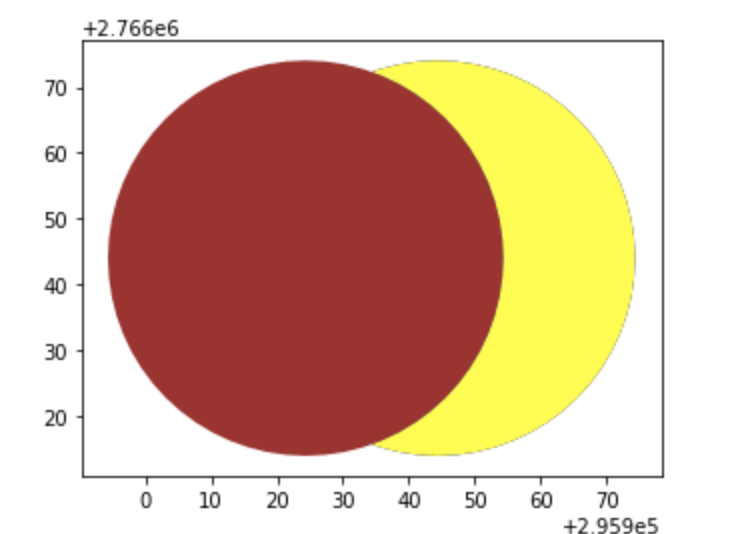### intersection

``````intersection = gpd.overlay(p1,p2,  how='intersection')
base=p1.plot(color='blue')
p2.plot(ax=base,color='brown')
intersection.plot(ax=base,color='yellow')
``````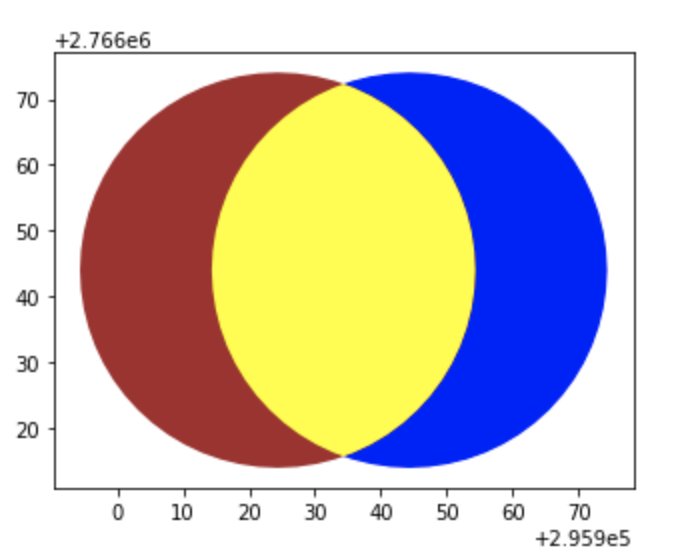### union

``````union = gpd.overlay(p1,p2,  how='union')
base=p1.plot(color='blue')
p2.plot(ax=base,color='brown')
union.plot(ax=base,color='yellow')
``````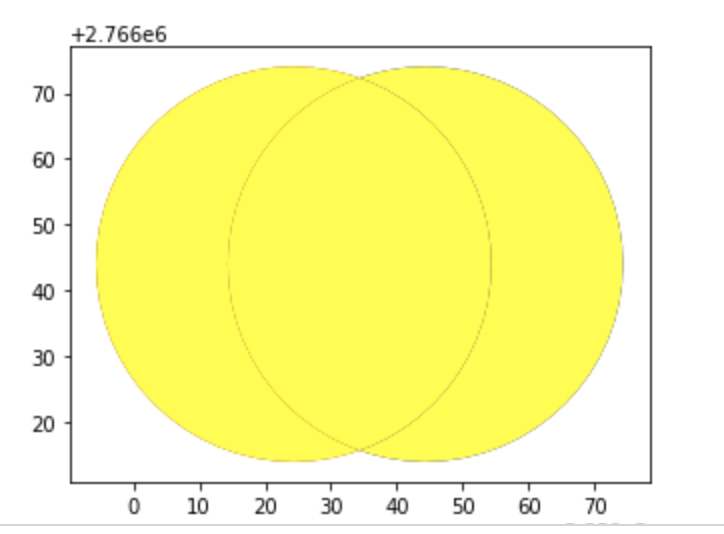### difference

difference會算出兩個幾何的差異，一樣以黃色表示

``````difference = gpd.overlay(p1,p2,  how='difference')
base=p1.plot(color='blue')
p2.plot(ax=base,color='brown')
difference.plot(ax=base,color='yellow')
``````